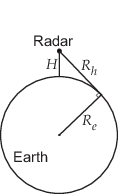# horizonrange

Horizon range

Since R2021a

## Syntax

``Rh = horizonrange(H)``
``Rh = horizonrange(H,Re)``
``Rh = horizonrange(___,SurfaceHeight=surfht)``

## Description

example

````Rh = horizonrange(H)` returns the horizon range, `Rh`, of a radar system `H` meters above the surface. The computation uses an effective earth radius of approximately 4/3 times the actual earth radius.```
````Rh = horizonrange(H,Re)` specifies the effective earth radius, `Re`.```
````Rh = horizonrange(___,SurfaceHeight=surfht)` also specifies the surface height, `surfht`.```

## Examples

collapse all

Find the range to the horizon from an antenna that is 30 m high.

`R = horizonrange(30)`

## Input Arguments

collapse all

Height of the radar system above the surface in meters, specified as a scalar or a vector.

Data Types: `double`

Effective earth radius in meters, specified as a positive scalar. You can use `effearthradius` to compute the effective radius. The function provides a default value approximately 4/3 times the actual earth radius

Example: `6.4e6`

Data Types: `double`

Height of earth surface at the horizon in meters, specified as a scalar or vector. This input can also be interpreted as the height of significant ground clutter at the horizon. If `H` and `surfht` are vectors, their lengths must be equal.

Data Types: `double`

## Output Arguments

collapse all

Horizon range in meters of radar system at altitude `H`, returned as a scalar or a vector.

collapse all

### Horizon Range

The horizon range of a radar system is the distance from the radar system to the earth along a tangent. Beyond the horizon range, the radar system detects no return from the surface through a direct path.The value of the horizon range is:

`$\sqrt{2{R}_{e}H+{H}^{2}}$`

where Re is the effective earth radius and H is the altitude of the radar system.

 Long, Maurice W. Radar Reflectivity of Land and Sea, 3rd Ed. Boston: Artech House, 2001.

 Skolnik, M. Introduction to Radar Systems, 3rd Ed. New York: McGraw-Hill, 2001.